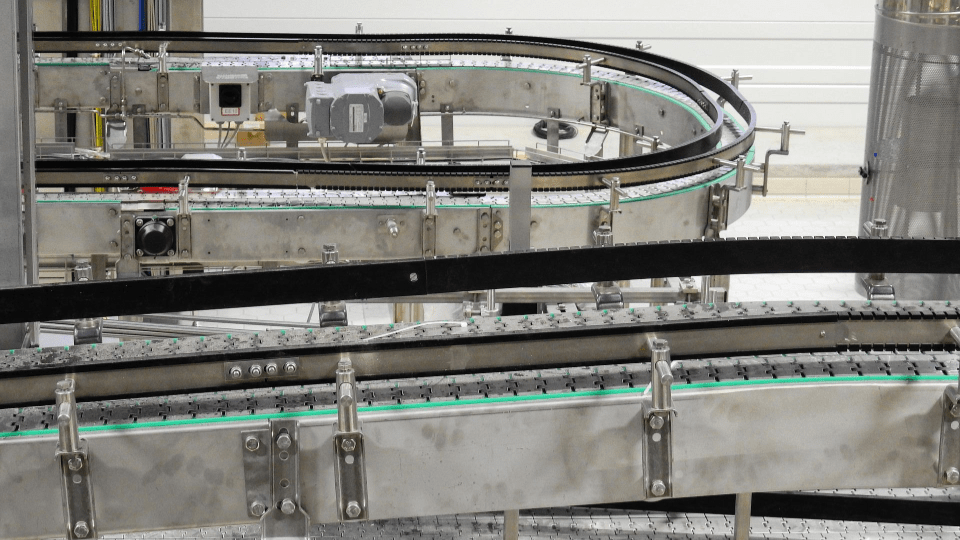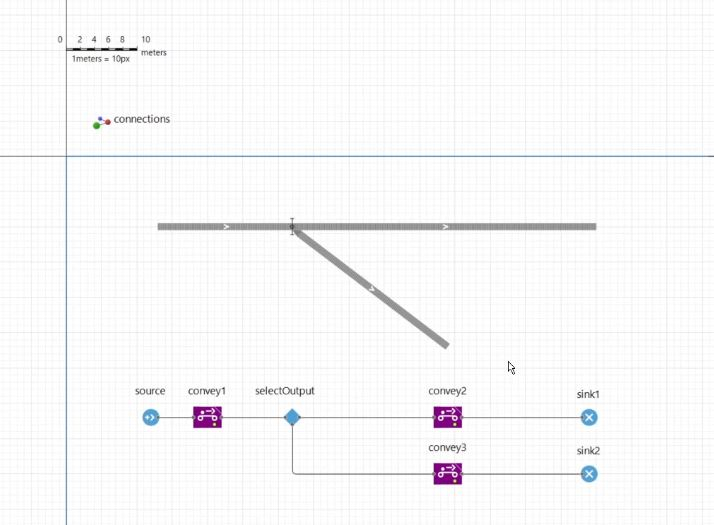# AnyLogic [EN]

## Conveyor routing simulation in AnyLogicHaving introduced AnyLogic with various smaller examples on this blog I here post a summary of a simulation model example published on The AnyLogic Modeler. I show how AnyLogic can be used for assessing conveyor layout variants. You can find […]

## AGV simulation of part routings in AnyLogicIn this article I present a simple AGV simulation model. It is meant as an example on how to simulate part routings in a factory. I implemented the simulation in AnyLogic. I have provided some other basic introductions to AnyLogic […]

## Queueing systems solved analyticallyUnderstanding the performance metrics of queueing systems is one of the areas where simulation has its strengths. In most operations planning, queues are considered wasteful and actions should be taken to reduce and minimize them. Queues at e.g. airports, train […]

## Conveyor simulation in AnyLogicThis post is the first post in an upcoming series of posts on AnyLogic simulation modelling. I want to introduce basic material handling system simulation modelling using AnyLogic as a discrete-event simulation machine and modelling environment. I will use AnyLogic’s […]